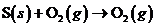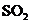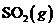Chapter 10, Problem 42QAP### Introductory Chemistry: A Foundati...

9th Edition
Steven S. Zumdahl + 1 other
ISBN: 9781337399425

#### Solutions

Chapter
Section### Introductory Chemistry: A Foundati...

9th Edition
Steven S. Zumdahl + 1 other
ISBN: 9781337399425
Textbook Problem
1 views

# For the reactionkJ per mole offormed. Calculate the quantity of heat released when 1.00 g of sulfur is burned in oxygen. Calculate the quantity of heat released when 0.501 mole of sulfur is burned in air. i>What quantity of energy is required to break up I mole ofinto its constituent elements?

Interpretation Introduction

(a)

Interpretation:

Calculate the heat produced when a specific amount of sulfur.

Concept Introduction:

Convert the number of moles for the provided mass of sulfur then calculate the heat produced per mole.

Explanation

Calculate heat produced for 1.00 g of sulfur as follows:

1.00g1molS32gS×1molSO

Interpretation Introduction

(b)

Interpretation:

Calculate the heat produced when a specific amount of sulfur.

Concept Introduction:

Use the moles of sulfur then calculate heat produced.

Interpretation Introduction

(c)

Interpretation:

Check the sign change for reverse reaction

Concept Introduction:

The enthalpy change for a reaction is equal in magnitude but opposite in sign, to the enthalpy change for the reverse reaction.

### Still sussing out bartleby?

Check out a sample textbook solution.

See a sample solution

#### The Solution to Your Study Problems

Bartleby provides explanations to thousands of textbook problems written by our experts, many with advanced degrees!

Get Started